# Python Program to Print ASCII Value

In this article, I've created some programs in Python, that find and prints ASCII value(s) in following ways:

• Print ASCII Value of single Character entered by User
• Print ASCII Values of all 255 Characters
• Print ASCII Values of all characters in a String entered by User

## Find and Print ASCII Value of a Character

This program find and prints ASCII value of a particular character entered by user at run-time. The ord() method returns ASCII value of a character passed as its argument, like shown in the program given below:

```print("Enter a Character: ")
ch = input()

asc = ord(ch)
print("\nASCII Value:", asc)```

Here is its sample run: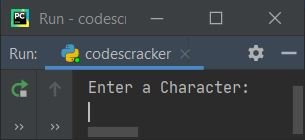Now supply the input say c and press `ENTER` key to find and print the ASCII value 'c' (entered character by user) as shown in the snapshot given below: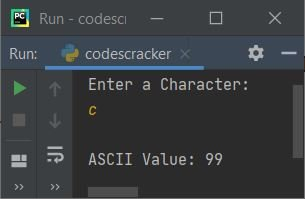#### Modified Version of Previous Program

The end skips inserting an automatic newline using print(). The str() converts any type of value to string type. The len() returns length of a string passed as its argument. The \" is used to print "

```print("Enter a Character: ", end="")
ch = input()

chlen = len(ch)
if chlen==1:
asc = ord(ch)
print("\nASCII Value of \"" +(ch)+ "\" = " +str(asc))
else:
print("\nInvalid Input!")```

Here is its sample run with user input Z: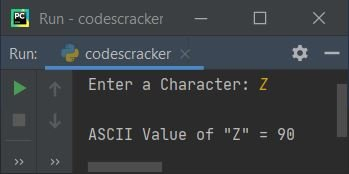Here is another sample run with user input codescracker. Since it is not a character, rather it is a string, therefore the program produces following output. At last of this article, I've created a program that prints ASCII value of anything like character, string, etc.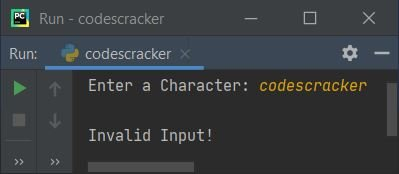## Print ASCII Values of all Characters

This program prints ASCII value of all 255 characters:

```print("ASCII\tCharacter")
for i in range(256):
ch = chr(i)
print(i, "\t\t", ch)```

The snapshot given below shows a small part of the output produced by above Python: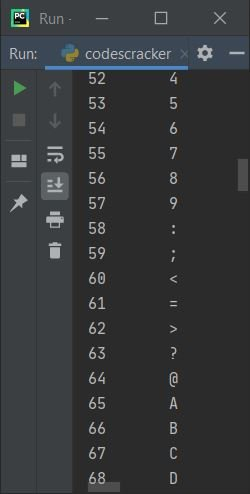The following code from above program:

`for i in range(256):`

states that the statements inside it gets executed 256 number of times with value of i from 0 to 255.

## Find and Print ASCII Value of all Characters in String

This program find and prints ASCII value of all characters in a string entered by user.

```print("Enter a String: ", end="")
text = input()

textlen = len(text)
for ch in text:
asc = ord(ch)
print(ch, "\t", asc)```

Here is its sample run with input, CodesCracker: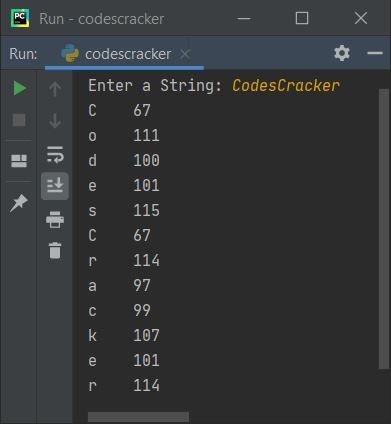Here is another sample run with user input as codes123%#4/|]{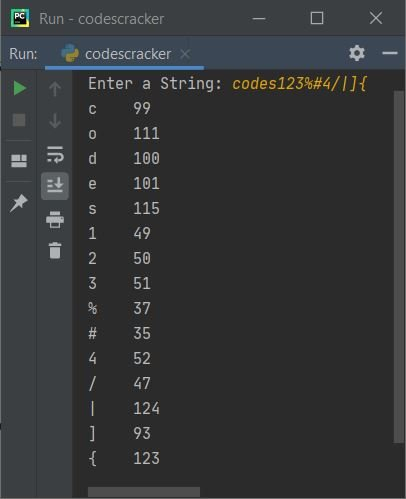#### Modified Version of Previous Program

The previous program is modified because that program prints ASCII value of c from string say codescracker three times. Since there are three c available in this string. But this program prints ASCII value of all characters for only one time, without mattering whether the character occurs one or more times in the string:

```print("Enter a String: ", end="")
text = input()

text = "".join(dict.fromkeys(text))
textlen = len(text)
for ch in text:
asc = ord(ch)
print(ch, "\t", asc)```

Here is its sample run with string user input as codescracker.com: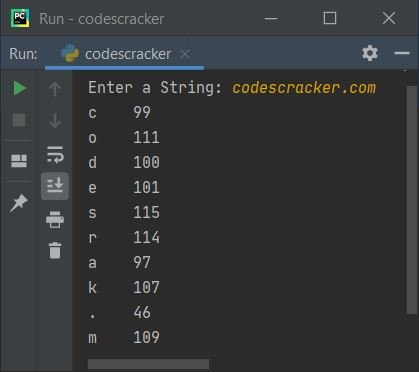Note - In above program, the statement `text = "".join(dict.fromkeys(text))`, removes duplicate characters from the string entered by user, stored in text.

Note - Dictionary does not allows duplicates.

#### Same Program in Other Languages

Python Online Test

« Previous Program Next Program »